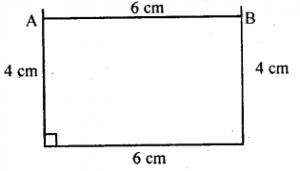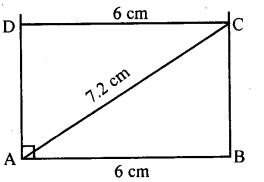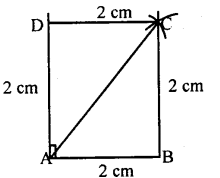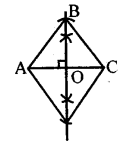# KSEEB Solutions for Class 8 Maths Chapter 15 Quadrilaterals Ex 15.5

In this chapter, we provide KSEEB SSLC Class 8 Maths Chapter 15 Quadrilaterals Ex 15.5 for English medium students, Which will very helpful for every student in their exams. Students can download the latest KSEEB SSLC Class 8 Maths Chapter 15 Quadrilaterals Ex 15.5 pdf, free KSEEB SSLC Class 8 Maths Chapter 15 Quadrilaterals Ex 15.5 pdf download. Now you will get step by step solution to each question.

### Karnataka State Syllabus Class 8 Maths Chapter 15 Quadrilaterals Ex 15.5

Question 1.
Construct rectangle ABCD with the following data.
(a) AB = 4 cm, BC = 6 cm.
Opposite sides of a rectangle are equal as each angle is a right angle.
∴ AB = CD = 4 cm.
Steps:1. Draw a line segment AB = 6 cm.
2. Construct a perpendicular at B.
3. With a 7 cm radius at A as center draw an arc on the perpendicular. Let the point be C.
4. With BC as radius draw an arc from A.
5. With AB as radius cut the arc from A.

(b) AB = 6 cm AC = 7.2 cm
Steps:1. Draw a line segment AB = 6 cm.
2. Draw perpendicular at B.
3. With a 4 cm radius cut the line at A.
4. With AD = 6 cm draw an arc.
5. With CD – 4 cm cut the arc.

Question 2.
Construct square ABCD
(a) Which has side length 2 cm.
(b) Which has diagonal 6 cm.
(a). Steps1. Draw a line segment AB = 2 cm.
2. At A, construct a perpendicular
3. With A as center cut the perpendicular
4. With a 2 cm radius from B and D draw the arc.
5. Join DC and BC.

(b) Steps:1. Draw a line segment AC = 6 cm.
2. Construct a perpendicular bisector to AC intersecting at O.
3. With O as the center and 3cm radius draw the line on both sides cutting at B and D.
4. Join AB, AD, DC & BC.

All Chapter KSEEB Solutions For Class 8 maths

—————————————————————————–

All Subject KSEEB Solutions For Class 8

*************************************************

I think you got complete solutions for this chapter. If You have any queries regarding this chapter, please comment on the below section our subject teacher will answer you. We tried our best to give complete solutions so you got good marks in your exam.

If these solutions have helped you, you can also share kseebsolutionsfor.com to your friends.

Best of Luck!!